×
Get Full Access to KSU - MKTG 35030 - Study Guide - Midterm
Get Full Access to KSU - MKTG 35030 - Study Guide - Midterm

×

KSU / Marketing / MKTG 35030 / How do costs change as volume changes?

# How do costs change as volume changes? Description

##### Description: Comprehensive study guide for Marketing Applications Exam 2, including: - GE-McKinsey Matrix - Response Functions - Four-Step Segmentation - Chocolates a la Carte Video Analysis - Product Philosophy - Product Dimensions - Seven-Step Segmentation Process - Anheuser-Busch Marketing Tactics
9 Pages 53 Views 1 Unlocks
Reviews

Introduction to Managerial Accounting [Acct-23021] Exam 1 Study Guide

## How do costs change as volume changes?Kent State University

Fall 2016

Chapter 6

I. Cost Behaviors

a. How costs change as volume changes

b. Variable Costs (VC)

i. Total VC change in direct proportion to changes in volume

ii. VC per unit remains constant

iii. Slope

1. Total VC = (VC per unit of activity) x (volume of activity)

2. Y=vx

c. Fixed Costs

i. Total fixed costs remains constant even if volume changes

ii. FC per unit of activity vary inversely with changes in volume 1. 500 desks: FC per unit = \$10,000/500 desks = \$20

## What is the meaning of committed fc?2. 600 desks: FC per unit = \$10,000/600 desks = \$16.67

3. 700 desks: FC per unit = \$10,000/700 desks = \$14.29

d. Mixed Costs

i. Combination of VC and FC

ii. Total mixed costs = total VC + total FC

1. Y = vx + f

e. Cost Graphs

i. Vertical axis always show total cost

ii. Horizontal axis shows volume of activityWe also discuss several other topics like What does a negative sustainable growth rate mean?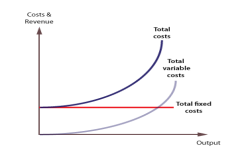## What is the meaning of discretionary fc?II. Cost Equations

a. Cost and Decisions

i. Committed FC – The business is locked in to these costs because of  previous management decisions

ii. Discretionary FC – Result of annual management decisions.

Companies have more control over discretionary FC because the  company can adjust the costs as necessary in the short run.

b. Relevant Range

i. Band of volume where FC and VC remain constant at a certain level  ii. A change in cost behavior indicates a change to a different relevant  range

c. Other Cost Behaviors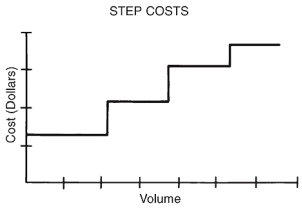Don't forget about the age old question of Why are immediate early genes important?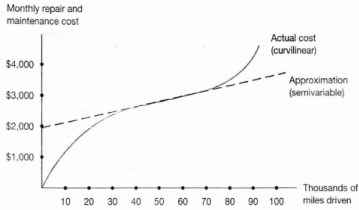Curvilinear CostsIf you want to learn more check out What is a process in which a perceived reality is organized in such a way that certain aspects of the reality are stressed?

III. Analyze Cost Behavior

a. Account Analysis

i. Managers use judgment to classify each general ledger account as a  VC, FC, or MC

ii. Estimates are subjective so it is not very reliable

b. Scatter Plots Don't forget about the age old question of According to the watson-crick model, dna exists in what form?

i. Use historical data to determine a cost’s behavior

ii. Cost data = y-axis

Volume Data = x-axis

iii. Helps managers visually determine how strong the relationship is  between the cost and the volume of the chosen activity base

IV. High-Low Method

a. Used as a cost analysis technique

b. Determine cost equation (y=vx+f)

i. Find VC per unit (slope) of cost line

ii. Find FC (vertical intercept)

iii. Create and calculate the cost equation

Disadvantage: Only uses 2 data points

V. Regression Analysis

a. Statistical procedure to find the line that best fits data (cost equation) b. Uses all data points

c. R-Square Value

i. “Goodness of fit”

ii. How well does this line fit the data points?

iii. Ranges from 0 to 1 If you want to learn more check out Why is the bonding molecular orbital of h2 at lower energy than the electron in a hydrogen atom?

1. An R-square over 0.80 generally indicates that the cost

equation is very reliable for predicting costs at other volumes

within the relevant range

2. 0.50-0.80 = Use the cost equation with caution

3. >0.50 = Use a different activity base for cost analysis

VI. Contribution Margin Income Statement

a. Absorption Costing (Traditional Income Statement)

i. Required by GAAP for external reporting

ii. Assign all manufacturing costs to products

1. DM, DL, Variable MOH, and Fixed MOH

b. Variable Costing

i. Assigns only variable manufacturing costs to products

1. DM, DL, and Variable Manufacturing Overhead

ii. Fixed MOH is a period cost

iii. Contribution margin income statements are used only for internal  management

c. Contribution Margin Income Statement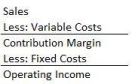We also discuss several other topics like What is the significance of a pyridoxine vitamin b6 deficiency?

Chapter 7

I. Cost-Volume-Profit (CVP) Analysis

a. Helps managers make important business decisions

b. A relationship between among costs, volume, and profit/loss

c. Determines how much the company must sell each month just to cover costs  or to break even

d. Components of CVP Analysis

i. Relies on the interdependency of five components

1. Sales price per unit

2. Volume sold

3. Variable costs per unit

4. Fixed costs

5. Profit or loss

II. Breakeven Points and Target Profit Volumes

a. Breakeven Point (BEP)

i. Sales level at which operating income is zero

1. Sales > BEP = Profit

2. Sales < BEP = Loss

b. Contribution Margin to Calculate BEP

i. BEP Units Sold = (Fixed Expenses + Operating Income) /

(Contribution Margin Per Unit)

1. (\$700 + \$0) / (\$14) = 500 units

ii. BEP Sales in \$ = (Fixed Expenses + Operating Income) /

(Contribution Margin Ratio)

1. (\$700 + \$0) / (0.4) = \$17,500

2. Contribution Margin Ratio = (Contribution Margin) / (Sales)

c. Finding volume needed for target profit

i. Same formulas but input amount for operating income

ii. Target profit = \$4,900

iii. BEP Units Sold

1. (\$700 + \$4,900) / (\$14) = 850 units

iv. BEP Sales in \$

1. (\$700 + \$4,900) / (0.4) = \$29,750

d. CVP Chart

i. Red Area = Operating Loss

ii. Green Area = Operating Income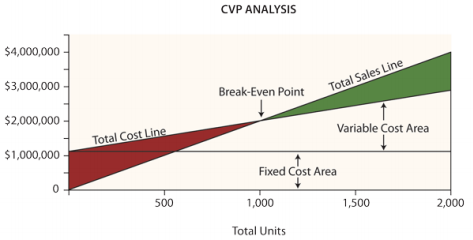III. BEP and Target Profit for Multiproduct Companies

a. Weighted Average Contribution Margin per Unit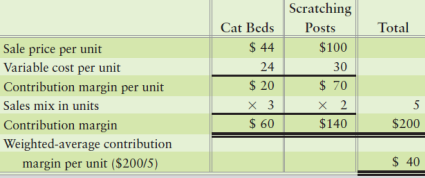b. Formula

i. Units Sold = (Fixed Expenses + Operating Income) / (Weighted  Avg. Contribution Margin per Unit)

ii. (\$7,000 + \$0)/(\$40) = 175 units

c. Breakeven sales

i. (Units)(percent of whole) = # of units per product to breakeven ii. 175 x 3/5 = 105 cat beds

iii. 175 x 2/5 = 70 scratching posts

IV. Margin of Safety, Operating Leverage, Most Profitable Cost Structure a. Margin of Safety

i. The excess of expected sales over breakeven sales

ii. Drop in sales that the company can absorb before incurring a loss iii. Used to evaluate the risk of current operations as well as the risk of  new plans

iv. Equations

1. Margin of Saftey (in units) =

Expected sales (in units) / Breakeven sales (in units)

2. Margin of Safety (in \$) =

Expected sales (in \$) / Breakeven sales (in \$)

3. Margin as a % of Expected Sales =

Margin of Safety (in \$) / Expected sales in (in \$)

b. Operating Leverage

i. The relative amount of fixed and variable costs that make up a  company’s total costs

ii. Operating Leverage = Contribution Margin / Operating Income iii. High operating leverage companies have:

1. Higher levels of FC and lower levels of VC

2. Higher contribution margin ratios

3. Higher risk

4. Higher potential for reward

iv. Low operating leverage companies have:

1. Higher level of VC and lower levels of FC

2. Lower contribution margin ratios

3. Lower risk

4. Lower reward

V. Miscellaneous Information

a. Contribution Margin = Revenue – VC

b. Contribution Margin Ratio = Contribution Margin / Sales Revenue

i. (Revenue – VC) / Revenue ~~ (this is the same thing)

c. Breakeven point is dependent on sales mix assumption

Chapter 8

I. Short-Term Decisions

a. Qualitative factors

i. Closing manufacturing plants

ii. Laying off employees

iii. Outsourcing

iv. Discounted prices to select customers

b. Six short-term special decisions

i. Special sales orders

ii. Pricing

iii. Discontinuing products, departments, and stores

iv. Outsourcing

v. Selling as is or processing further

c. Keys to making short-term special decisions

i. Only look at how operating income will or will not change under  different options

ii. FC and VC react differently so they must be analyzed separately II. Special Order Considerations

a. Is there excess capacity available to fill the order?

b. Is the reduced sales price high enough to cover the incremental costs of  filling the order?

c. Will the special order affect regular sales in the long run?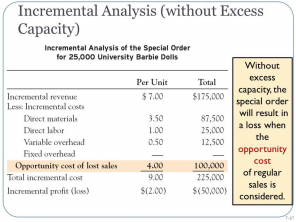III. Different Approaches to Pricing

a. Target Costing (Price-taker)

i. Market price – desired profit = target cost

ii. Characteristics

1. Product lacks uniqueness

2. Heavy competition

iii. Outcomes

1. Actual cost < Target total cost

a. This is okay. Proceed with product price

2. Actual cost > Target total cost

a. Accept a lower profit

b. Cut FC

c. Cut VC

d. Other strategies

i. Increase sales volume

ii. Change or add product mix

iii. Differentiate

Revenue @ Market Price

\$205,000

Less: Desired Profit

29,120

Predetermined % (16%) of VC (182,000)

Target Cost per Unit

175,880

Less: Actual Current VC

182,000

Total Variable Costs

Expected Excess Profit (profit shortfall)

(6,120)

b. Cost-Plus Pricing (Price-setter)

i. Starts with company’s full costs

ii. Add desired profit to determine cost-plus price

Current Variable Costs

\$182,000

Total VC

32,000

Total VC

214,000

New VC

Plus: Desired Profit

34,240

Predetermined % of VC (16%)

Cost-Plus Price

248,240

IV. Discontinuing Products, Departments, etc.

a. Will the total FC continue to exist even if the product line is discontinued? b. Can any direct FC of the product be avoided if the product is discontinued? c. Use incremental analysis for discontinuing a product

d. Will dropping the product affect sales of the company’s other products? e. What can be done with the freed capacity?

V. Which product to emphasize?

a. Emphasize the product with the highest contribution margin per unit of the  constraint

b. In addition to revenues, the additional costs of transforming product 1 in to  other products must be considered

c. The cost of processing raw materials is irrelevant since it will be incurred in  all products offered

Product 1

Product 2

Product 3

Revenue

12,000

101,000

200,000

0

68,000

175,000

Net Benefit

12,000

34,000

25,000

VI. Analyze Outsourcing Decisions

a. Compare costs

i. Which VC are cheaper?

ii. Are any FC avoidable if we outsource?

iii. What could we do with the freed capacity

Direct material

0.46

Direct labor

0.74

0.22

Variable cost per unit

1.42

1.04

Full (absorption) cost per unit

2.46

VII. Selling As-Is or Processing Further

a. How much revenue is generated if we sell as-is?

b. How much revenue is generated if we process further?

c. How much will it cost to process further

Page ExpiredIt looks like your free minutes have expired! Lucky for you we have all the content you need, just sign up here